### Curve Fitting: Linear Regression

### Linear Regression

Given a set of data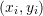with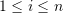, a linear model fit to this set of data has the form: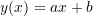whereandare the model parameters. The model parameters can be found by minimizing the sum of the squares of the difference (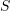) between the data points and the model predictions: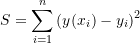Substituting for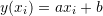yields: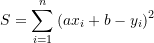In order to minimize, we need to differentiatewith respect to the unknown parametersandand set these two equations to zero: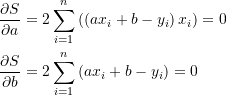Solving the above two equations yields the best linear fit to the data. The solution forandhas the form: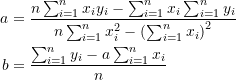The built-in function “Fit” in mathematica can be used to find the best linear fit to any data. The following code illustrates the procedure to use “Fit” to find the equation of the line that fits the given array of data:

View Mathematica Code
Data = {{1, 0.5}, {2, 2.5}, {3, 2}, {4, 4.0}, {5, 3.5}, {6, 6.0}, {7,  5.5}};
y = Fit[Data, {1, x}, x]
Plot[y, {x, 1, 7}, Epilog -> {PointSize[Large], Point[Data]}, AxesLabel -> {"x", "y"}, AxesOrigin -> {0, 0}]

View Python Code
import numpy as np
import matplotlib.pyplot as plt

Data = [[1, 0.5], [2, 2.5], [3, 2], [4, 4.0], [5, 3.5], [6, 6.0], [7,  5.5]]
p = np.polyfit([point for point in Data],
[point for point in Data], 1)
x = np.linspace(1, 7)
y = np.poly1d(p)
plt.plot(x, y(x))
plt.title(y)
plt.scatter([point for point in Data],[point for point in Data], c='k')
plt.xlabel("x"); plt.ylabel("y")
plt.grid(); plt.show()


#### Coefficient of Determination

The coefficient of determination, denoted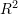or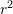and pronounced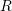squared, is a number that provides a statistical measure of how the produced model fits the data. The value ofcan be calculated as follows: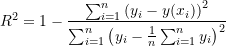The equation above implies that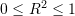. A value closer to 1 indicates that the model is a good fit for the data while a value of 0 indicates that the model does not fit the data.

#### Using Mathematica and/or Excel

The built-in Mathematica function “LinearModelFit” provides the statistical measures associated with a linear regression model, including the confidence intervals in the parametersand. However, these are not covered in this course. The “LinearModelFit” can be used to output theof the linear fit as follows:

View Mathematica Code
Data = {{1, 0.5}, {2, 2.5}, {3, 2}, {4, 4.0}, {5, 3.5}, {6, 6.0}, {7, 5.5}};
y2 = LinearModelFit[Data, x, x]
y = Normal[y2]
y3 = y2["RSquared"]
Plot[y, {x, 1, 7}, Epilog -> {PointSize[Large], Point[Data]}, AxesLabel -> {"x", "y"}, AxesOrigin -> {0, 0}]

View Python Code
import numpy as np
from scipy import stats
import matplotlib.pyplot as plt

Data = [[1, 0.5], [2, 2.5], [3, 2], [4, 4.0], [5, 3.5], [6, 6.0], [7,  5.5]]
p = np.polyfit([point for point in Data],
[point for point in Data], 1)
print("p: ",p)
slope, intercept, r_value, p_value, std_err = stats.linregress([point for point in Data],
[point for point in Data])
print("R Squared: ",r_value**2)
y = np.poly1d(p)
print("y:",y)
x = np.linspace(1, 7)
plt.title(y)
plt.plot(x, y(x))
plt.scatter([point for point in Data],[point for point in Data], c='k')
plt.xlabel("x"); plt.ylabel("y")
plt.grid(); plt.show()


The following user-defined Mathematica procedure finds the linear fit andand draws the curve for a set of data:

View Mathematica Code
Clear[a, b, x]
Data = {{1, 0.5}, {2, 2.5}, {3, 2}, {4, 4.0}, {5, 3.5}, {6, 6.0}, {7, 5.5}};
LinearFit[Data_] :=
(n = Length[Data];
a = (n*Sum[Data[[i, 1]]*Data[[i, 2]], {i, 1, n}] - Sum[Data[[i, 1]], {i, 1, n}]*Sum[Data[[i, 2]], {i, 1, n}])/(n*Sum[Data[[i, 1]]^2, {i, 1, n}] - (Sum[Data[[i, 1]], {i, 1, n}])^2);
b=(Sum[Data[[i, 2]], {i, 1, n}] - a*Sum[Data[[i, 1]], {i, 1, n}])/    n;
RSquared=1-Sum[(Data[[i,2]]-a*Data[[i,1]]-b)^2,{i,1,n}]/Sum[(Data[[i,2]]-1/n*Sum[Data[[j,2]],{j,1,n}])^2,{i,1,n}];
{RSquared,a,b })
LinearFit[Data]
RSquared=LinearFit[Data][]
a = LinearFit[Data][]
b = LinearFit[Data][]
y = a*x + b;
Plot[y, {x, 1, 7}, Epilog -> {PointSize[Large], Point[Data]}, AxesLabel -> {"x", "y"}, AxesOrigin -> {0, 0} ]

View Python Code
import numpy as np
import matplotlib.pyplot as plt

Data = [[1, 0.5], [2, 2.5], [3, 2], [4, 4.0], [5, 3.5], [6, 6.0], [7, 5.5]]
def LinearFit(Data):
n = len(Data)
a = (n*sum([Data[i]*Data[i] for i in range(n)]) - sum([Data[i] for i in range(n)])*sum([Data[i] for i in range(n)]))/(n*sum([Data[i]**2 for i in range(n)]) - sum([Data[i] for i in range(n)])**2)
b = (sum([Data[i] for i in range(n)]) - a*sum([Data[i] for i in range(n)]))/n
RSquared = 1 - sum([(Data[i] - a*Data[i] - b)**2 for i in range(n)])/sum([(Data[i] - 1/n*sum([Data[j] for j in range(n)]))**2 for i in range(n)])
return [RSquared, a, b]
x = np.linspace(0,7)
LinearFit(Data)
print("LinearFit(Data): ",LinearFit(Data))
RSquared = LinearFit(Data)
print("RSquared: ",RSquared)
a = LinearFit(Data)
b = LinearFit(Data)
print("a: ",a)
print("b: ",b)
y = a*x + b;
plt.plot(x, y)
plt.scatter([point for point in Data],[point for point in Data], c='k')
plt.xlabel("x"); plt.ylabel("y")
plt.grid(); plt.show()


Microsoft Excel can be used to provide the linear fit (linear regression) for any set of data. First, the curve is plotted using “XY scatter”. Afterwards, right clicking on the data curve and selecting “Add Trendline” will provide the linear fit. You can format the trendline to “show equation on chart” and.

#### Example

Find the best linear fit to the data (1,0.5), (2,2.5), (3,2), (4,4), (5,3.5), (6,6), (7,5.5).

##### Solution

Using the equations for,, and: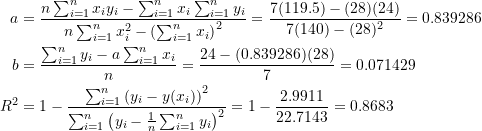Microsoft Excel can easily be used to generate the values for,, andas shown in the following sheet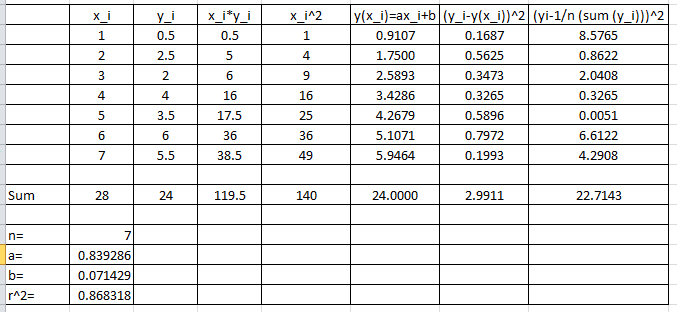The following is the Microsoft Excel chart produced as described above.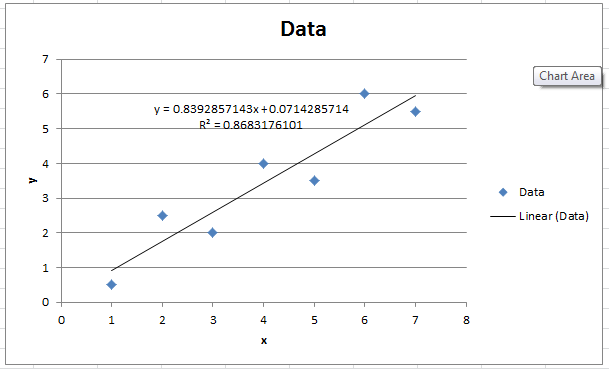You can upload some data on the online Python app on mecsimcalc that performs these calculations which is embedded below as well.

The following link provides the MATLAB code for implementing the linear curve fit.

MATLAB file: